# E1-15 Suppose the following items were taken from the balance sheet of Nike, Inc. (Alldollars are in millions.)7. Inventory8. Income taxes payable9. Equipment10. Retained earnings11. Accounts payable\$2,357.0\$2,291.12. Accounts receivable 2,883.92,874.21. Cash86.33. Common stock1,957.75,818.92,815.84. Notes payable5. Buildings6. Mortgage payable342.93,759.91,311.5InstructionsPerform each of the following.(a) Classify each of these items as an asset, liability, or stockholders' equity, and deter-mine the total dollar amount for each classification.(b) Determine Nike's accounting equation by calculating the value of total assets, totalliabilities, and total stockholders' equity.(c) To what extent does Nike rely on debt versus equity financing?

Question
80 views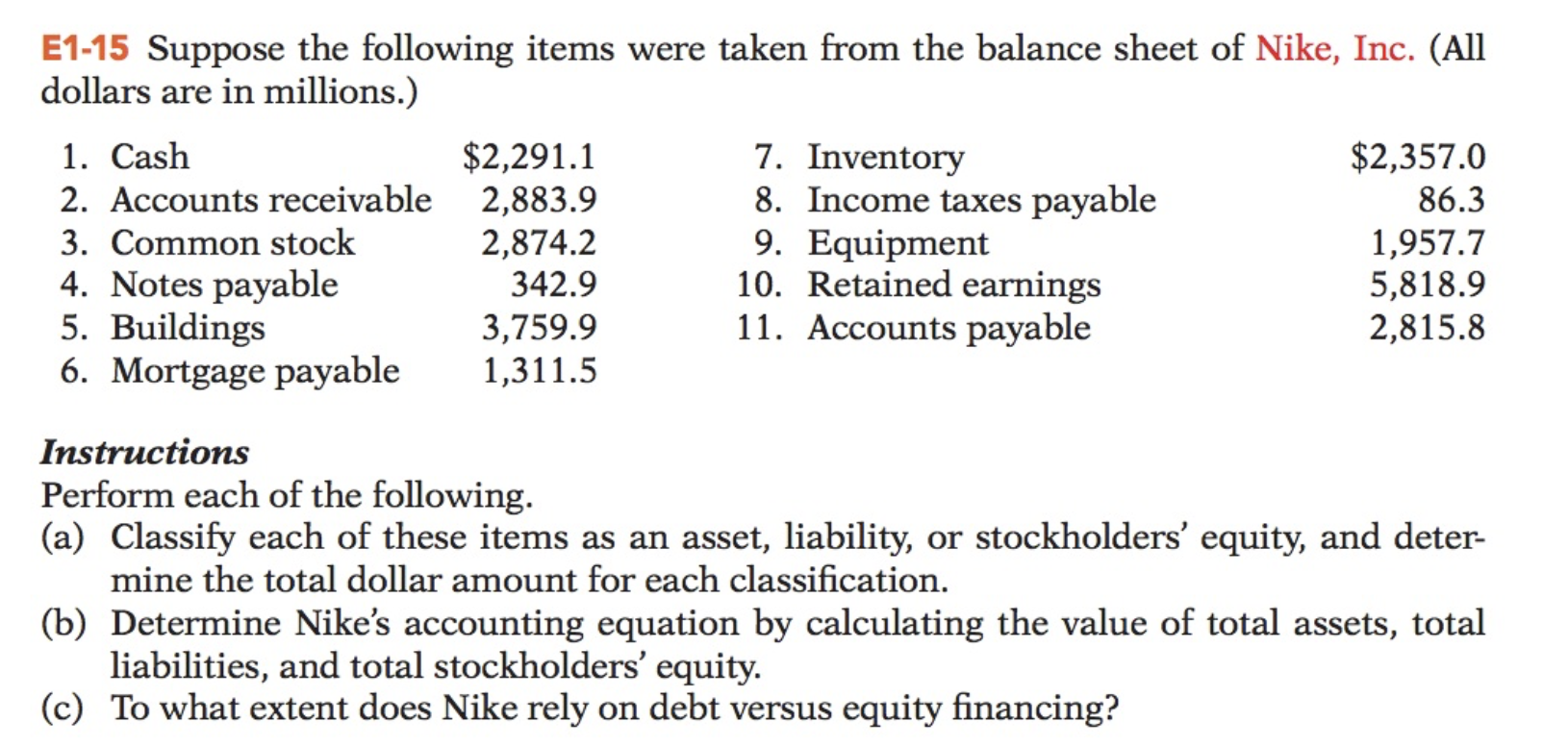help_outlineImage TranscriptioncloseE1-15 Suppose the following items were taken from the balance sheet of Nike, Inc. (All dollars are in millions.) 7. Inventory 8. Income taxes payable 9. Equipment 10. Retained earnings 11. Accounts payable \$2,357.0 \$2,291.1 2. Accounts receivable 2,883.9 2,874.2 1. Cash 86.3 3. Common stock 1,957.7 5,818.9 2,815.8 4. Notes payable 5. Buildings 6. Mortgage payable 342.9 3,759.9 1,311.5 Instructions Perform each of the following. (a) Classify each of these items as an asset, liability, or stockholders' equity, and deter- mine the total dollar amount for each classification. (b) Determine Nike's accounting equation by calculating the value of total assets, total liabilities, and total stockholders' equity. (c) To what extent does Nike rely on debt versus equity financing? fullscreen
check_circle

a. Classify and calculate the total of the items asset, liabilities or stockholder's equity.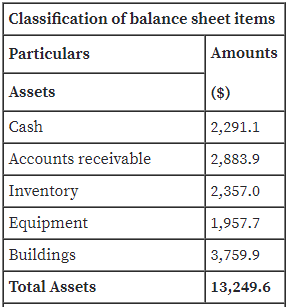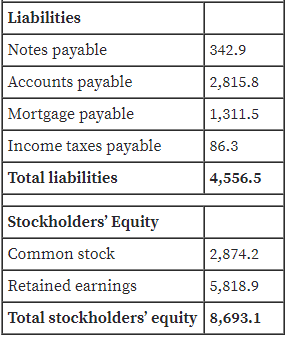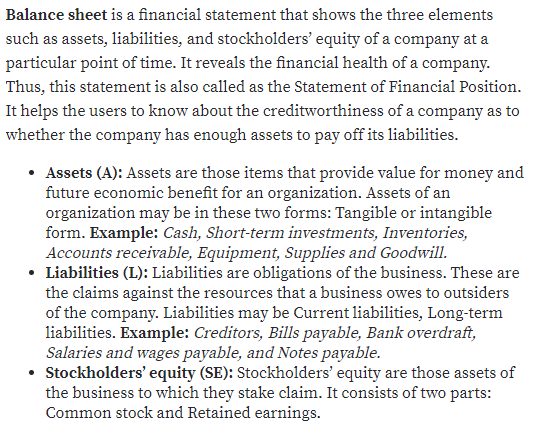b. Determine the accounting equation.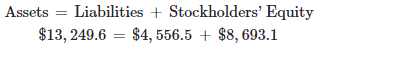...

### Want to see the full answer?

See Solution

#### Want to see this answer and more?

Solutions are written by subject experts who are available 24/7. Questions are typically answered within 1 hour.*

See Solution
*Response times may vary by subject and question.
Tagged in